#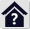Friction brakes and clutches

## Friction brakes and clutches

The program is intended for the design, calculation and validation of four basic types of friction brakes and clutches. It also allows the user to determine the kinetic energy and loading moments of the mechanisms.

2. Calculation of clutch starting load
3. Validation of a loaded clutch
4. Design and validation of:
- Disc brakes/clutches
- Cone brakes/clutches
- Drum brakes/clutches
- Band brakes/clutches
5. Calculation and temperature validation of the designed brake / clutch

The calculation uses data, procedures, algorithms and data from specialised literature, standards and company catalogues.

 Clutches and Brakes Design and Selection;  Shigley's Mechanical Engineering Design;  Textbook of Machine Design;  Brake Design and Safety;  Roloff / Matek - Maschinenelemente
VDI 2241-Blatt 1; VDI 2241-Blatt 2; SAE J866; SAE J661; Ortlinghaus, Goizper, TAROX, FERODO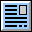User interface.

Purchase, Price list.

## Control, structure and syntax of calculations.

Information on the syntax and control of the calculation can be found in the document "Control, structure and syntax of calculations".

## Information on the project.

Information on the purpose, use and control of the paragraph "Information on the project" can be found in the document  "Information on the project".

## Theory

### Foreword

The friction brakes and clutches do not differ in physical nature. The difference is only in the waythe brake/clutch is loaded, their geometric design and control. The specific solution and the corresponding calculation also correspond to this. The given relationships are universal.

### The principle of the solution

The solution of a brake/clutch calculation usually consists of two related tasks.

1. Calculation of the brake/clutch load based on the braking/running mechanism - paragraph  in the Calculation.
2. Calculation of brake/clutch dimensions to meet the required load - paragraphs ,,, and  in the Calculation.

#### Brake - Braked mechanism

The braked mechanism has a kinetic energy Ek at the beginning of the braking cycle (It depends on the square of the speed.).
- Rotational motion
- Rectilinear motion

And at the same time, it is subject to forces and moments that decrease/increase the brake load (they are constant during the braking cycle).
- Bearing frictional forces
- External influence (braking on an inclined plane)
- Aerodynamic drag (not constant) etc.

Braking time is an another variable.

The solution to these relationships is the torque that the brake must develop and the energy that must be absorbed by the brake.

#### Clutch - starting mechanism

For the clutch, the analogous procedure applies as it does for the brake (starting the mechanism -> reaching the desired Ek).

It is possible to separate the two tasks and to obtain, for example, the basis for the selection of the brake/clutch from the manufacturer's catalogue, or to design the brake/clutch on the basis of the load.

The following paragraphs contain formulas for load calculation, energy calculation, formulas used for friction brake and clutch dimensioning and temperature calculations.

### Energy calculations

#### Kinetic energy

Kinetic energy is calculated as the sum of the kinetic energy of straight-line motion and the energy of rotation of the mass.

Ek [J]= 0.5 * m * v2 + 0.5 * I * ω2

m ... mass of the braked mass [kg]
v .... velocity of the braked mass [m/s]
I .... moment of inertia of the braked mass [kg*m²]
ω ... speed of the braked mass with moment of inertia I [rad/s]

When braking or starting a mechanism, there are usually additional forces or moments that must be included in the calculation. These can be wheel rolling resistance, bearing rolling resistance, forces due to mass (crane, lift, movement on an inclined plane), friction, aerodynamic resistance, etc.
All these additional forces and moments must then be converted to brake/clutch speed by the appropriate gear ratio.

For example, if the vehicle wheel speed is nw=100 [rpm] and the clutch speed is nc=2000 [rpm], the additional ML moment applied to the clutch is:

ML = Mw * nw / nc

Mw ... moment applied to the vehicle wheel
nw ... wheel speed
nc .... clutch speed

#### Reduced moment of inertia

With multiple rotating and moving parts with different speeds, it is useful to add their kinetic energies and convert them to a reduced moment of inertia based on brake/clutch speed for further calculations.

Ired [kg*m²] = 2 * Ek / (n / 60 * 2 * p)2

Ek ... sum of kinetic energies [J)
n ..... brake/clutch speed [/min]

### Coefficient of friction

The coefficient of friction is the basic parameter that most influences the brake/clutch dimensions. It depends on the materials used and their combination. In the Calculation, it is possible to choose from the basic areas of friction materials. However, we strongly recommend that you follow the specific values of the manufacturer or supplier.

Example of how the coefficient of friction f [~] can vary depending on pmax [MPa], v [m/s] and T [°C] for dry friction for the material combination Powdered metal / steel.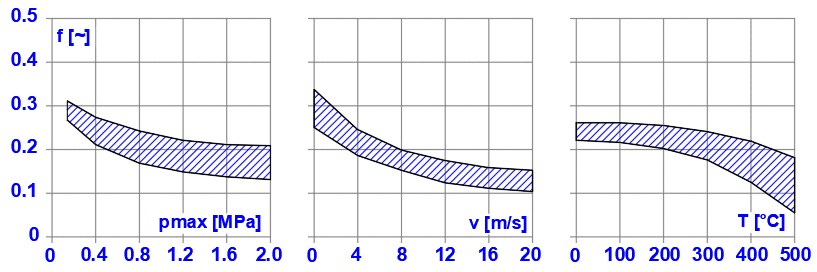### Disc brakes/clutches

Disc brakes and disk clutches are some of the most common types of brakes and clutches. They have simple construction, large friction surface area, and good heat dissipation. It is possible to use designs with one or more friction surfaces, especially when the design with 2 friction surfaces is significantly used in the automotive industry (disc brakes, disc clutches).Since the principle of brakes and couplings is not different for the calculation, a common procedure is used with the dimensions defined by the figure below. Brakes are usually designed in such a way that the friction element is an annular sector pad or circle (A, B) and the clutch is an annular contact (C).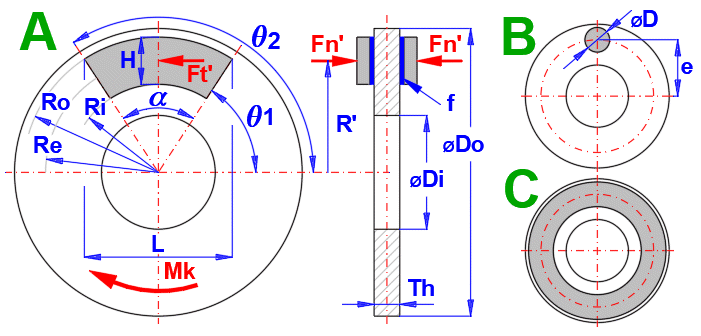Two approaches are used for the solution.

A. Uniform wear (run-in brake/clutch): It is based on the assumption that the friction discs are rigid and the greatest wear occurs first on their outer circumference, where the work of frictional forces is the greatest. After some wear occurs, the pressure distribution changes, so that the wear of the friction surfaces is even.

B. Uniform pressure (new brake/clutch): It assumes that by using springs that evenly press the friction discs into contact, a uniform pressure is achieved over the entire friction surface.

Generally, approach A is used.

#### Uniform wear (run-in lining)

Maximum pressure
pmax [MPa] =(2 * Mk) / (a * f  * Re * (Ro^2 - Ri^2) / 1000000 / FillCoeff

Re [m] = (Ro + Ri) / 2

Friction force
Ft [N] = Mk / Re

Normal force
Fn [N] = Ft / f

Point of action of the normal force
R' [m] = (COS(q1) - COS(q2)) / (q2 - q1) * (2/3) * ((Ro^3 - Ri^3) / (Ro^2 - Ri^2))

#### Uniform pressure (new lining)

Maximum pressure
pmax [MPa] = (3 * Mk) / (a * f * (Ro^3 - Ri^3)) / 1000000 / FillCoeff

Re [m] = 2 / 3 * (Ro^3 - Ri^3) / (Ro^2 - Ri^2)

Friction force
Ft [N] = Mk / Re

Normal force
Fn [N] = Ft / f

Point of action of the normal force
R' [m] = (COS(q1) - COS(q2)) / (q2 - q1) * ((Ro + Ri)/2)
a ..................... Friction segment angle [rad]
f ....................... Friction coefficient [~]
FillCoeff ............ Filling coefficient [~]

### Cone brakes/clutches

A conical surface is used as a friction surface. The advantage of this design is a lower actuation force Fn than a disc brake/clutch. Depending on the friction material, a cone angle b in the range of 10 -15° is usually used.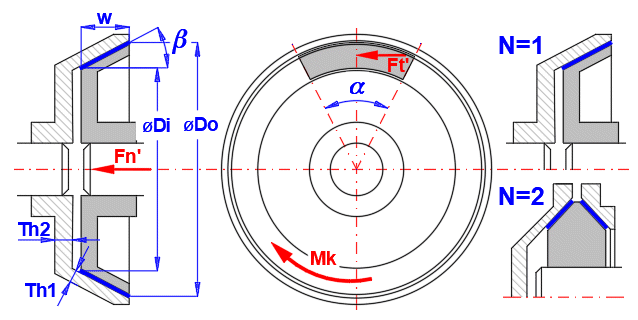The solution is carried out similarly to the previous case, by determining the relations that are valid for uniform wear of the lining and for uniform distribution of pressure over the entire friction surface.

#### Uniform wear (run-in lining)

Maximum pressure
pmax [MPa] = (Mk / (p * f * Di) * (8 * SIN(b)) / (Do^2 - Di^2)) / 1000000 / a

Equivalent diameter De
De [m]=(Do + Di) / 2

Friction force
Ft [N] = Mk / (De / 2)

Normal force
Fn [N] = Ft * SIN(b) / f

#### Uniform pressure (new lining)

Maximum pressure
pmax [MPa] = (Mk /(p * f) * (12 * SIN(b)) / (Do^3 - Di^3)) / 1000000 / (a / (2 * p))

Equivalent diameter De
De [m] = 2/3 * (Do^3 - Di^3) / (Do^2 - Di^2)

Friction force
Ft [N] = Mk / (De / 2)

Normal force
Fn [N] = Ft * SIN(b) / f

a ...... Friction segment angle [rad]
f ....... Friction coefficient [~]
Do .... Outer diameter [m]
Di ..... Inner diameter [m]

### Drum brakes/clutches

Drum brakes with internal shoes are mainly used in the automotive industry. Outer shoe brakes and symmetric shoe brakes are used mainly in industrial applications.In principle, two types of shoes are used in drum brakes. Depending on the sense of rotation (torque applied), they are referred to as Leading shoe R and Trailing shoe L, as they are marked in the figure L, R.
For the same force F, the braking moments, pressures, forces and reactions are different for the shoe (L) and (R).

The calculation is therefore principally carried out in the following steps (leading (R) and trailing (L) shoe):
===============================================================
1. Calculation of the force F' for unit pmaxR'=1 for the leading shoe (R)
2. Calculation of the braking moment MR' for the leading shoe (R) for the force F'
3. Calculation of pmaxL' for the left shoe(L) for the force F'
4. Of which the calculation of the braking moment for the left shoe (L) ML'
5. Calculation of the total braking torque Msum' = MR'+ML'
6. Calculation of the coefficient X, X = Mk / Msum'
7. Conversion of unit values of pressures, forces, moments and reactions by the coefficient X

#### Relationships used in the calculation:

Frictional moment
Mt_R [Nm] = f * pmax * w * (D/2) / SIN(qXa) * CoefA
CoefA =(D / 2 * (-(COS(qX2) - COS(qX1))) - a * ((0.5 * SIN(qX2)^2) - (0.5*SIN(qX1)^2)))/1000

Normal moment
Mn_R [Nm] = pmax * w * (D/2) * a / SIN(tqXa) * CoefB / 1000
CoefB =(qX2/2 - 1/4 * SIN(2 * qX2)) - (qX1/2 - 1/4 * SIN(2 * qX1))

Force F
F [N] = (Mn_R - Mt_R) / (c / 1000)
c [mm] = ABS(y1 - y2)

Braking moment M_R
M_R [Nm] = f * pmax * w * (D/2)^2 * (COS(qX1) - COS(qX2)) / SIN(qXa) / 1000

Pressure pmaxL
pmaxL [MPa] = (F * c / (Mn_R + Mt_R)) / 1000

Braking moment M_L
M_L [Nm] = f * pmaxL * w * (D/2)^2 * (COS(qX1) - COS(qX2)) / SIN(qXa) / 1000

Braking moment Msum
Msum [Nm] = NR * M_R + NL * M_L

X coeficient
X = Mk / Msum

f ............. Friction coefficient [~]
pmax ...... Maximum pressure [MPa]
w ........... Shoe face width [mm]
D ........... Drum diameter [mm]
qX1 = q1 - a1 [rad]
qX2 = q2 - q1 + qX1 [rad]
qXa ... a) qX2 < PI()/2 ... qXa = qX2, b) qX2 > PI()/2 ... qXa = PI()/2
NR, NL ... Number of braking elements (leading (R) and trailing (L) shoe )

### Brakes with symmetrically mounted shoesThe distance "a" of the axis of the shoe pin from the axis of the drum, which ensures zero friction torque to the axis of the shoe pin. This ensures symmetrical wear of the lining.

a = 4 * (D/2) * SIN(q1') / (2 * q1' + SIN(2 * q1'))
q1' = pi()/2 - q1

Maximum pressure
pmax [Mpa] = (Mk / (NL+NR)) / (2 * f * w  * (D / 2)^2 * SIN(q1')) / FillCoeff

Reaction in the pin of the shoe in the x and y direction
Rx = pmax * w * (D/4) * (2 * q1' + SIN(2 * q1'))
Ry = pmax * w * f * D/4 * (2 * q1' + SIN(2 * q1'))

where:
q1 ............... brake lining start angle (see figure) [rad]
NL, NR ......... number of braking elements [~]
f .................. coefficient of friction [~]
w ................. width of braking element [m]
D ................. outer diameter of drum [m]
q1' ............... half belt angle [rad]
FillCoeff ........ filling coefficient [~]

### Band brakes/clutches

The friction surface consists of a belt that is wrapped around the brake drum. It is used in industrial applications, simple design. It is suitable for manual operation when high braking performance can be achieved with relatively low operating force.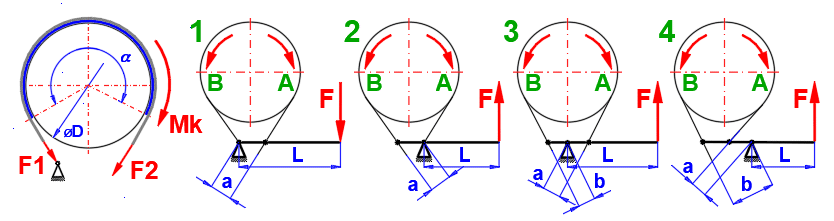#### Relations used in the calculation:

ecoef = 2.7182818 (f *a)
Ft = Mk / ((Do / 2))
F2 = Mk / (ecoef - 1) * (Do / 2))
F1 = Ft + F2

Maximum pressure
pmax = (2 * F1 / (w * Do)) / N

Calculation of the force F

A: in the direction of rotation:
1 ... F = (Ft * a / L) * (1 / (ecoef - 1)) / N
2 ... F = (Ft * a / L) * (ecoef / (ecoef - 1)) / N
3 ... F = (Ft / L) * ((b * ecoef - a) / (ecoef - 1)) / N
4 ... F = (Ft / L) * ((b * ecoef + a) / (ecoef - 1)) / N

B: against the direction of rotation:
1 ... F = (Ft * a / L) * (ecoef / (ecoef - 1)) / N
2 ... F = (Ft * a / L) * (1 / (ecoef - 1)) / N
3 ... F = (Ft / L) * ((b - a * ecoef) / (ecoef - 1)) / N
4 ... F = (Ft / L) * ((b + a * ecoef) / (ecoef - 1)) / N

f ........... Friction coefficient [~]
Ft .......... Friction force [N]
Do ......... Drum outer diameter [m]
a .......... Drum belt angle [rad]
N .......... Number of belts [~]
a,b,L ..... Lever dimensions [mm]

### Calculation of warming

During both braking and starting, kinetic energy is converted into heat when the friction surfaces come into contact. The preceding paragraphs give the relationships for calculating the maximum torque and maximum pressure on the friction surfaces for the specified brake/clutch dimensions. Even if the maximum torque is not transmitted and the maximum allowable pressure is not exceeded, the brake/clutch may be destroyed by excessive heat.

#### Assumptions for warming calculation

This calculation obviously does not cover all brake/clutch designs. (oil cooling, special geometries, special materials, etc.). Therefore the following assumptions are used:
- The friction lining has a low thermal conductivity l compared to the thermal conductivity of the materials in contact with the lining. (l for friction lining ~ 0.1, l for steel ~ 54). Therefore, it is assumed that all the heat generated by friction is absorbed by the metal part of the brake/clutch
- The thermal properties of the materials are constant (In the real case, of course, they change, although not significantly)
- Braking time is an order of magnitude shorter than cooling time
- The weight of the clutch/brake (It is only an estimate. It is not a substitute for a detailed analysis of the mass of the body receiving the heat generated by friction)

#### Heat flux density through the surface S''

Some manufacturers also specify a maximum heat flux value q for friction segments.
q [W/mm²] = Eh / t / S''

Eh ..... The heat energy generated by the clutch/brake during the working cycle [J].
t ........ Time of braking/clutch engagement [s]
S''' ...... Frictional area of disc/drum [mm²]

#### Warming up (disc, drum, housing...) for one-time braking / clutch actuation

The temperature rise for the brake/clutch can be approximately determined by the formula:
ΔT1 [°C] = Eh / (c * m)

Eh ... The thermal energy generated by the clutch/brake operation during the duty cycle [J].
c ..... Specific heat capacity of the material [J/kg/K]
m .... Mass of the clutch/brake [kg]
As the formula does not take into account the time factor and cooling, it should be taken as a guide.

#### Friction surface warming for one-time braking / clutch actuation

Calculation of contact surface warming
ΔT2 [°C] = (5 / 18)^0.5 * (Eh * t^0.5) / (S'' * t * (Ro * c * l)^0.5)

Eh .......... Thermal energy generated by the clutch/brake during the duty cycle [J]
Ro .......... Density [kg/m^3]
c ............ Specific heat capacity of the material [J/kg/K]
l ........... Thermal conductivity of the material [W/m/K]
t ............ Braking/clutch engagement time [s]
S'' .......... Frictional area of disc/drum [m^2]

Heat transfer time tb to reach the outer surface of the drum
tb [s] = Th^2 / (5 * (l / (Ro * shc)))

Th .......... Drum/disc thickness [mm]
c ............. Heat capacity of the material [J/kg/K]

#### Warming with repeated use

The previous equation ΔT1 [°C] = Eh / (c * m) can be used together with Newton's cooling law, which describes the heat transfer from the clutch/brake surface.

After appropriate simplification and the use of simplifying assumptions, we obtain this equation:

(T - TA) / (T1 -TA) = exp(- (a * S) / (m * c) * t)

T ..... The surface temperature of the body at time t [°C].
TA ... Temperature of the immediate surroundings of the surface of the body - air temperature [°C]
T1 ... Initial temperature of the surface of the body [°C]
a .... The heat transfer coefficient by flow and radiation [W*m^-2/K]
S ..... External surface area of the body [m²]
m .... Mass of the body (brake, clutch)
c ..... Specific heat capacity of the material [J/kg/K]
t ..... Cooling time [s]

For repeated use, the braking process can then be described as shown in the figure below: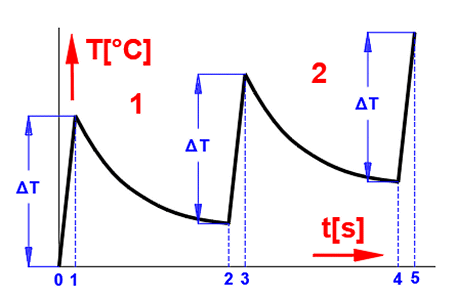0-1 ... Braking of the mechanism - frictional energy absorption, temperature increase
1-2 ... Transfer of energy to the environment (cooling - temperature drop)
2-3 ... Next braking cycle, temperature increase
3-4 ... Transfer of energy to the environment (cooling - temperature drop)

..................

The temperature development for a repeated cycle can then look like this.When the temperature of the brake/clutch rises, the heat transfer coefficient a increases accordingly, so that at a certain temperature the energy input (braking process) and the energy output (cooling) are balanced.

#### Heat transfer coefficient a

The heat transfer coefficient a in the case of this calculation is influenced by 3 parameters and the following relation is used for its calculation:

a = aR + aC * fv

aR .... Radiative heat transfer coefficient [W*m^-2/K]
aC .... Heat transfer coefficient by flow [W*m^-2/K]
fv ...... Air flow coefficient [~]

The following graph can be used to determine the coefficients aR and aC:
dT = T - TA

T ..... The surface temperature of the body at time t [°C]
TA ... Temperature of the immediate surroundings of the body surface - air temperature [°C]To determine the Air flow coefficient fv then: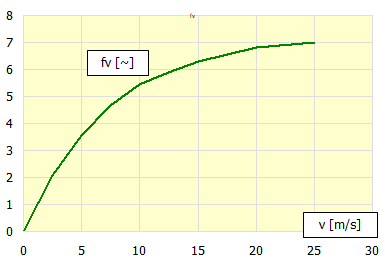#### Iterative calculation

This calculation simulates 100 steps of repeated braking/clutch engagement with a specified time interval. For each step, the corresponding heat transfer coefficient alpha is calculated, which is then used to calculate the temperatures in the next step. In most cases, this procedure is satisfactory and converges quickly to a steady state temperature.

#### Cumulative calculation

In this calculation, you estimate the total temperature increase. From this increase, the corresponding heat transfer coefficient alpha is calculated, which is used for the overall warming calculation. This procedure is dependent on the estimate of warming and may be less accurate than the previous calculation. However, if the previous procedure does not converge to a steady state end temperature, this calculation should be used.
Example of a warming trend where it is appropriate to use the cumulative calculation.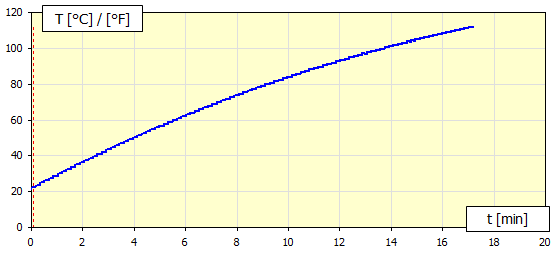## Calculation procedure:

The solution of the brake/clutch calculation is usually divided into two tasks.

1. Calculation of the brake/clutch load based on the braked/actuated mechanism .
2. Calculation of brake/clutch dimensions to meet the required load [2,3,4,5].

###### Note: The dimensional design of a friction brake or clutch is physically identical. The only differences are in the moments and energies captured/transferred by the brake/clutch.

In paragraph 1.0, select the method of load calculation (brake/clutch) [1.3].
Enter or calculate the kinetic energy Ek and the moment ML (additional calculations [6,7]) and enter the speed, time and operating parameters.
The result is the required moment and energy sufficient for the supplier's brake/clutch specification or for the dimensional calculations in paragraphs [2,3,4,5].

#### 2. Calculation of dimensions

After selecting the brake/clutch type [2,3,4,5], enter the torque applied to the brake/clutch.
Enter the friction surface parameters and friction element dimensions.
The result is the friction surface pressure, speed and applied forces.

By linking the calculation of the load  and the calculation of the dimensions [2,3,4,5], it is then possible to solve other problems.
- Brake/clutch temperature during operation
- Optimization of dimensions

## Units, calculation of energies, loading moments, selection of materials and coefficients 

In this paragraph you set the calculation units and calculate the load torque and energy required for the correct design of the brake/clutch dimensions.
Here you also set other parameters such as friction material properties, brake/clutch material and load type.

### 1.1 Calculation units.

Select the desired system of calculation units in the list box. After switching over the units, all values will be changed immediately.

### 1.2 Method of calculating energy and load

To calculate brakes/clutches, it is usually necessary to know "what we are braking" or "what we want to move". This information leads to two basic parameters.
1. The torque that the brake/clutch must transmit
2. The energy the brake/clutch must absorb

Thus, there are three basic calculations of energy and torque.
A. Brake calculation
B. Starting clutch calculation
C. Running clutch check

The selected calculation is active, the remaining two are greyed out - inactive.
The geometric calculation of the specific brake/clutch type [2,3,4,5] then takes the information about the load moment, energy, etc. from the selected energy and moment calculation; as well as the brake/clutch warming calculation 

### 1.3 Brake calculation

The task is to brake the moving mechanism to the required state (usually to a stop n2=0).
In this case, we solve the problem where the kinetic energy Ek and the additional moments ML must be converted into thermal energy in the friction brake.

Used assumptions and simplifications:
- All braked masses are interconnected at constant transmission
- The load moment ML is constant during the braking process

### 1.4 Initial kinetic energy of the mechanism

It is the kinetic energy of the moving masses you need to brake. To calculate them, you can use the paragraphs [6.0], where you can switch with the "Ek >>" button.

It is a load/unload moment (+ / -) converted to brake speed n1.

To solve common tasks, press the "ML >>" button.

- Vehicle movement downhill
- Elevator, crane (mass applied force)

- Vehicle movement uphill
- Rolling resistance of the mechanism

### 1.6 Reduced moment of inertia

It is a moment of inertia converted from all braked masses (rotational, linear motion - see Ek calculation) to the initial speed of the braked disc (drum) n1.

### 1.7 Initial speed of the brake disc (drum)

Enter the initial speed of the brake disc or drum.

### 1.8 Brake disc (drum) end speed

Enter the end speed of the brake disc or drum. For a complete stop of the braking mechanism n2 = 0.

### 1.9 Required braking time

It is a required braking time from speed n1 to n2.

### 1.10 Theoretical braking torque

It is a theoretically calculated torque (assuming optimum conditions) that the brake must provide during the braking cycle.

### 1.11 Service coefficient

Since the braking mechanism may exhibit non-uniformity (torque oscillation), it is advisable to use a coefficient (KA) that takes this unevenness into account and guarantee the required braking time. The design of the KA coefficient is based on practical knowledge and its size is governed by the setting of the conditions on line [1.41].

You can enter your own value by unchecking the check button.

### 1.12 Torque for brake design

It is the torque that the brake must capture. This torque value is used to calculate the brake dimensions [2,3,4,5].

### 1.13 Brake heating energy

It is the amount of energy that must be dissipated in the brake (brake warming).

### 1.14 Calculation of the clutch (starting)

The task is to run the mechanism to the desired state (usually from n2=0 to n1=required speed). In this case, we solve the problem when it is necessary to supply to the mechanism such energy Ek, which is necessary to achieve the required speed and revolutions while overcoming the additional moments ML.

Used assumptions and simplifications:
- The speed n1 (drive) is constant during the clutch operation
- A constant moment is transmitted => the acceleration of the mechanism is constant

### 1.15 Final kinetic energy of the mechanism

It is the kinetic energy to which the mechanism must be accelerated. You can use paragraphs  for the calculation, where you can switch with the "Ek >>" button.

Load / unload moment (+ / -) related to the clutch speed n1.

To calculate the usual tasks, press the "ML >>" button.

- Starting the vehicle uphill
- Rolling resistance of the mechanism
- Elevator, crane (mass applied by force)

- Starting the vehicle downhill

### 1.17 Reduced moment of inertia

Moment of inertia converted from all expanding masses (rotational, linear motion - see Ek calculation) to the initial speed of the clutch disc (drum) n1.

### 1.18 Drive power (electric motor, internal combustion engine ...)

The power of the driving machine that is supplied to the clutch.

### 1.19 Clutch speed (drive side)

Enter the speed of the clutch on the drive side. The mechanism will be accelerated to this speed.

### 1.20 Clutch speed (mechanism side)

In most cases, you will be dealing with starting a stopped mechanism (n2=0).

If you need to solve a mechanism that is already in motion (n2>0) and accelerate it to speed n1, enter the initial speed n2.

### 1.21 Drive torque

It is the torque delivered by the driving machine.

### 1.22 Service coefficient

Since both the driven mechanism and the driving machine may exhibit non-uniformity (torque oscillation), it is convenient to use the coefficient (KA). This coefficient takes this non-uniformity into account when calculating the torque that the coupling must transmit.

The design of the KA coefficient is based on practical knowledge and its size is governed by the setting of the conditions in line [1.40, 1.41 and 1.42].

To enter your own value, uncheck the check button.

### 1.23 Torque for clutch design

It is the torque that the clutch must transmit. This torque value is used to design the dimensions of the coupling [2,3,4,5].

### 1.24 Clutch efficiency

The generally reported efficiency of friction clutch is approximately 0.95.

### 1.25 Torque available to achieve Ek

This is the portion of torque that is supplied by the driving machine and is fully utilized to achieve the desired final Ek of the mechanism.
The other part of the torque is dissipated in the clutch in the form of friction.
The moment Mu is reduced/increased by the value of ML [1.16].

### 1.26 Clutch engagement time (mechanism start-up)

The required clutch operation time is calculated (value in the green field) based on:
- The required end kinetic energy of the mechanism Ek [1.15]
- Engine power Pw [1.18]
- Clutch efficiency h [1.24]

To enter your own clutch operation time, uncheck the check button. Then the value Ek '[1.27], to which the mechanism is accelerated, corresponds to the entered time.

### 1.27 Actual end kinetic energy achieved

It is the kinetic energy of the mechanism that is achieved by engaging the clutch in the time specified in the previous line.

### 1.28 Energy for the temperature rise of the clutch

It is the energy that is converted to heat in the clutch.

### 1.29 Clutch check (constant load)

When solving the energy transfer through a friction clutch, it is advisable to check the functionality under operating conditions.
The clutch may be suitable for starting the mechanism (see previous calculation - starting) but may not be suitable for steady state energy transfer when the operating parameters are reached.

Example:
The clutch will ensure the mechanism starts at n1 at the specified power Pw.
The driving machine, with the clutch engaged, further increases the power Pw and the speed n to the operating values.
The clutch must ensure torque transmission even under operating conditions.

### 1.30 Drive power (electric motor, internal combustion engine ...)

The power of the driving machine that is supplied to the clutch.

### 1.31 Speed

Enter the operating speed of the clutch at which the corresponding power is taken.

### 1.32 Drive torque

It is the torque delivered by the driving machine.

### 1.33 Service coefficient

Since both the driven mechanism and the driving machine may exhibit non-uniformity (torque oscillation), it is convenient to use the coefficient (KA). This coefficient takes this non-uniformity into account when calculating the torque that the coupling must transmit.

The design of the KA coefficient is based on practical knowledge and its size is governed by the setting of the conditions in line [1.40, 1.41 and 1.42].

To enter your own value, uncheck the check button.

### 1.34 Torque for clutch design

It is the torque that the clutch must transmit. This torque value is used to design the dimensions of the coupling [2,3,4,5].

### 1.35 Preliminary design of shaft diameters (steel)

This paragraph gives designs of shaft diameters (steel) which correspond to the desired loading (Mk). These values are orientation values only; it is advisable to use a more exact calculation for the final design.

### 1.39 Design of the KA coefficient

When designing a brake/clutch, the exact load moment curves are usually not known.

Therefore, the KA coefficient is used in the calculations to tell, based on experience and testing, how to increase the torque so that the designed brake/clutch can handle the appropriate load nonuniformity.

For the brake calculation, the coefficient KA is suggested based on the type of braking mechanism.
To calculate the clutch, the coefficient KA is suggested, which includes both the braked mechanism and the drive machine and the operating time.

### 1.40 Driving machine

Select the appropriate type of driving machine from the list.

### 1.41 Driven / Braked mechanism, load

Working machine

Generators, chain conveyors, centrifugal, compressors, sand blasting blowers, textile machines, conveyor systems, fans and centrifugal pumps.

Elevators, bucket conveyors, rotary kilns, wire winders, crane travel and trolley drives, winches, agitators, shears, machine tools, washing machines, looms, brick extruders

Excavators, drilling rigs, briquetting presses, mine ventilators, rubber rolling mills, hoisting drives, pug mills, reciprocating pumps, tumblers, joggers, combination mills

Reciprocating compressors, frame saws, wet-presses, paper mangles, roller conveyors, drying rolls, roller mills, cement mills, centrifuges

### 1.43 Selection of friction material

The list contains a basic list of used friction materials. The coefficient of friction and the maximum allowable pressure and application are given in brackets.

Based on the selected material, the values [1.45-1.49] are pre-filled.

### 1.50 Selection of brake/clutch material (disc, drum, housing....)

Select a material that absorbs the energy dissipated when braking or starting the mechanism.

The selection has no influence on the calculation of the brake/clutch parameters. The material values are used to calculate the warming (weight).

Examples:
1. The disc brake absorbs the braking energy and is made of steel.
2. The clutch housing absorbs the loss energy and is made of aluminum.

## Disc brakes / clutches 

Disc brakes and disk clutches are some of the most common types of brakes and clutches. They have simple construction, large friction surface area, and good heat dissipation. It is possible to use designs with one or more friction surfaces, especially when the design with 2 friction surfaces is significantly used in the automotive industry (disc brakes, disc clutches).### 2.1 Basic input values

Two basic parameters are required for the calculation and design of the brake/clutch. Torque Mk and friction coefficient f.
These parameters, together with the definition of the dimensions, lead to the calculation of the maximum pressure on the friction surface, the calculation of the actuating force and, knowing the energy and time parameters from , the calculation of the heating.

### 2.2 Braking / transfer torque

In the green field is the moment Mk from paragraph . If you only need to solve the geometry for the specified torque Mk, uncheck the button on the right and enter the Mk to be transmitted by the brake/clutch. This breaks the connection with paragraph  and thus the warming and speed are not calculated (the results are grayed out).

### 2.3 Friction coefficient

By unchecking the check button on the right you can enter a custom value.
The recommended value is based on the selected friction material [1.44].

### 2.4 Friction segment definition

In this section you define the dimensions of the friction element. You can check the dimensions in the figure on the right.

### 2.5 Shape of the friction segment

There are three most common shapes to choose from:
A. Annular sector pad - mostly used for brakes.
B. Circular (Button or Puck) - use for less stressed brakes (easy to manufacture, simple design).
C. Annular contact - mostly used for clutches.

Depending on the choice of shape, the requirements for the respective input parameters are set.

### 2.6 Calculation method

Two approaches are used for the solution.

A. Uniform wear (run-in brake/clutch): It is based on the assumption that the friction discs are rigid and the greatest wear occurs first on their outer circumference, where the work of frictional forces is the greatest. After some wear occurs, the pressure distribution changes, so that the wear of the friction surfaces is even.

B. Uniform pressure (new brake/clutch): It assumes that by using springs that evenly press the friction discs into contact, a uniform pressure is achieved over the entire friction surface.

Generally, approach A is used.

### 2.7 Number of friction surfaces

Enter the number of friction surfaces according to your design (see picture).### 2.8 Outer radius of the friction segment / Distance of the centre of the circular friction segment

For the shape of the friction segment [2.5] A and C, enter: Outer radius of the friction segment Ro (see picture).

For shape B, enter: Distance of the centre of the circle "e" from the axis (see picture).

### 2.9 Height of friction segment / Diameter of circular friction segment

On the right, the optimum value of H (or D for a circular segment) is proposed to achieve the maximum friction torque from the relation Ri = Ro / 1.732.

You can enter your own value by unchecking the check button on the right.

### 2.11 Segment angle

For the friction segment shape [2.5] A, enter the segment angle (see picture).

Based on the area characteristic of the friction segment, the equivalent radius Re is calculated, which is used to calculate the forces.

### 2.13 Radius of action of the normal force Fn'

It is the radius at which the normal force Fn' should act.

### 2.16 Fill factor

Brake (clutch) linings are often attached with rivets. This coefficient expresses the reduction of the friction surface of the lining by the respective mounting holes.

For glued linings cF = 1.0 and for riveted linings cF ~ 0.90 - 0.95.

### 2.17 Contact area of one friction segment

It is the area over which the friction segment abuts the friction surface. It depends on the definition of the friction segment dimensions.

### 2.18 Sliding area for the friction segment

It is the area of the brake/clutch, which the friction segment is in contact with (indicated by a dashed line if visible on the outline).

### 2.19 Values for one friction surface

This section gives the values for one friction surface.

### 2.20 Braking / transmission torque

Total moment Mk divided over the individual friction surfaces.

### 2.23 Friction speed

Most friction material manufacturers also state the maximum value of friction speed that the material can handle. The recommended value is based on the choice of material [1.43].

### 2.24 Maximum pressure

It is the maximum pressure at the most heavily loaded point of the lining. Lining manufacturers usually state its maximum value (see recommended value on the right). If the recommended maximum value is exceeded, try to modify the geometry or the type of lining.

### 2.25 Heat flux density through the surface S''

Some manufacturers also provide a maximum recommended heat flux value q for friction segments.

### 2.26 Approximate temperature rise

The friction lining has a poor thermal conductivity lambda compared to the thermal conductivity of the materials in contact with the lining. (lambda of friction lining ~ 0.1, lambda for steel ~ 54). Therefore, it is assumed that all heat generated by friction is absorbed by the metal part of the brake/clutch.

This indicative calculation uses only the weight of the disc (cone, drum) m. Ssee the calculation below. The calculation is correct if the disc is the energy recipient (typically a disc brake).

For other design arrangements (typically a clutch), the frictional energy is absorbed, for example, by the clutch housing, and the result is obviously invalid.

### 2.27 Friction surface warming

It is assumed that the braking/clutch engagement process is short. Therefore, no cooling during this process is considered. The calculation of the friction surface warming is based on this assumption, where only the heat transfer to the disc (drum, housing, etc.) is considered based on the thermal conductivity of the material.

### 2.28 Finding a solution (GoalSeek)

Problems like:
- How to change the dimensions to reach the allowed pmax
- How can the brake/clutch be loaded to achieve the allowed pmax
- What can be the maximum force Fn'
and others can be easily solved using the excel function "GoalSeek".

For the most common tasks the inputs to this function and its call are predefined.

After selecting the query and entering the desired value, the "Run" button will start the function execution.

You can return to the original value of the changed parameter by pressing the "Back" button. (The functionality is time-limited to prevent unwanted overwriting of values).

###### Note: The function does not control the inputs and results of the solution. Therefore, it is possible that no solution exists for the specified request. In this case, set the original value of the modified parameter and re-evaluate your requirements.

Example:
You want to calculate the friction segment angle for a disc brake that will provide the required maximum pressure "pmax". It is clear that the friction segment angle can only be within a certain range, but there is no limit to the pmax requirement. So a requirement for a large change in pmax can lead to a completely meaningless alpha segment angle.

### 2.29 Selection of the requirement

Select the problem you want to solve from the list.

### 2.30 Required parameter value

Enter the desired value to be reached by changing the input parameter and call the "Solution search" function using the button on the right.

### 2.31 Brake disc calculation

The dimensional parameters are estimated on the basis of the previous data. After unchecking the check button on the right, you can enter your own dimensions (see figure).

The mass value is used to calculate ΔT1.

The area value is used for the cooling calculation in paragraph .

### 2.36 Surface

The value of surface S' is used as a default for the calculation of warming in paragraph  and is not used in this paragraph.

The area S' is calculated according to the figure.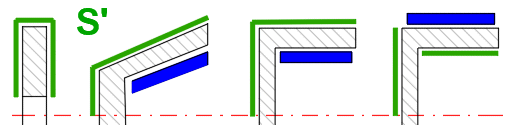## Cone brakes / clutches 

A conical surface is used as a friction surface. The advantage of this design is a lower actuation force Fn than a disc brake/clutch. Depending on the friction material, a cone angle b in the range of 10 -15° is usually used.### 3.1 Basic input values

Two basic parameters are required for the calculation and design of the brake/clutch. Torque Mk and friction coefficient f.
These parameters, together with the definition of the dimensions, lead to the calculation of the maximum pressure on the friction surface, the calculation of the actuating force and, knowing the energy and time parameters from , the calculation of the heating.

### 3.2 Braking/transfer torque

In the green field is the moment Mk from paragraph . If you only need to solve the geometry for the specified torque Mk, uncheck the button on the right and enter the Mk to be transmitted by the brake/clutch. This breaks the connection with paragraph  and thus the warming and speed are not calculated (the results are grayed out).

### 3.3 Friction coefficient

By unchecking the check button on the right you can enter a custom value.
The recommended value is based on the selected friction material [1.44].

### 3.4 Friction segment definition

In this section you define the dimensions of the friction element. You can check the dimensions in the figure on the right.

### 3.5 Shape of friction segment

There are two options to choose from:
A. Section of the conical surface - this construction is used rarely
B. Complete conical surface - used for brakes and clutches

Depending on the choice of shape, the requirements for the respective input parameters are set.

### 3.6 Calculation method

Two approaches are used for the solution.

A. Uniform wear (run-in brake/clutch): It is based on the assumption that the friction discs are rigid and the greatest wear occurs first on their outer circumference, where the work of frictional forces is the greatest. After some wear occurs, the pressure distribution changes, so that the wear of the friction surfaces is even.

B. Uniform pressure (new brake/clutch): It assumes that by using springs that evenly press the friction discs into contact, a uniform pressure is achieved over the entire friction surface.

Generally, approach A is used.

### 3.7 Number of friction surfaces

Enter the number of friction surfaces according to your design (see picture).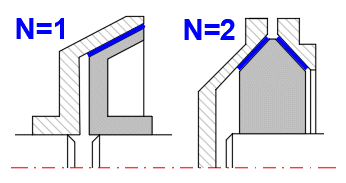### 3.8 Outer diameter of the conical surface

Enter the dimensions of the friction segment as shown.

### 3.11, 3.12 Cone angle / Cone width

You can specify the cone angle or the cone width. Use the switch on the right to set the option.

### 3.13 Equivalent diameter

Based on the area characteristic of the friction segment, the equivalent diameter De is calculated, which is used to calculate the forces.

### 3.14 Contact area of one friction segment

It is the area over which the friction segment abuts the friction surface. It depends on the definition of the friction segment dimensions.

### 3.15 Sliding area for the friction segment

It is the area of the brake/clutch, which the friction segment is in contact with (indicated by a dashed line if visible on the outline).

### 3.16 Values for one friction surface

This section gives the values for one friction surface.

### 3.17 Braking / transmission torque

Total moment Mk divided over the individual friction surfaces.

### 3.20 Friction speed

Most friction material manufacturers also state the maximum value of friction speed that the material can handle. The recommended value is based on the choice of material [1.43].

### 3.21 Maximum pressure

It is the maximum pressure at the most heavily loaded point of the lining. Lining manufacturers usually state its maximum value (see recommended value on the right). If the recommended maximum value is exceeded, try to modify the geometry or the type of lining.

### 3.22 Heat flux density through the surface S''

Some manufacturers also provide a maximum recommended heat flux value q for friction segments.

### 3.23 Approximate temperature rise

The friction lining has a poor thermal conductivity lambda compared to the thermal conductivity of the materials in contact with the lining. (lambda of friction lining ~ 0.1, lambda for steel ~ 54). Therefore, it is assumed that all heat generated by friction is absorbed by the metal part of the brake/clutch.

This indicative calculation uses only the weight of the disc (cone, drum) m. Ssee the calculation below. The calculation is correct if the disc is the energy recipient (typically a disc brake).

For other design arrangements (typically a clutch), the frictional energy is absorbed, for example, by the clutch housing, and the result is obviously invalid.

Note: The calculation includes one braking/running cycle. For a more detailed analysis use paragraph .

### 3.24 Friction surface warming

It is assumed that the braking/clutch engagement process is short. Therefore, no cooling during this process is considered. The calculation of the friction surface warming is based on this assumption, where only the heat transfer to the disc (drum, housing, etc.) is considered based on the thermal conductivity of the material.

### 3.25 Finding a solution (GoalSeek)

Problems like:
- How to change the dimensions to reach the allowed pmax
- How can the brake/clutch be loaded to achieve the allowed pmax
- What can be the maximum force Fn'
and others can be easily solved using the excel function "GoalSeek".

For the most common tasks the inputs to this function and its call are predefined.

After selecting the query and entering the desired value, the "Run" button will start the function execution.

You can return to the original value of the changed parameter by pressing the "Back" button. (The functionality is time-limited to prevent unwanted overwriting of values).

###### Note: The function does not control the inputs and results of the solution. Therefore, it is possible that no solution exists for the specified request. In this case, set the original value of themodified parameter and re-evaluate your requirements.

Example:
You want to calculate the friction segment angle for a disc brake that will provide the required maximum pressure "pmax". It is clear that the friction segment angle can only be within a certain range, but there is no limit to the pmax requirement. So a requirement for a large change in pmax can lead to a completely meaningless alpha segment angle.

### 3.26 Selection of the requirement

Select the problem you want to solve from the list.

### 3.27 Required parameter value

Enter the desired value to be reached by changing the input parameter and call the "Solution search" function using the button on the right.

### 3.28 Friction cone calculation

The dimensional parameters are estimated on the basis of the previous data. After unchecking the check button on the right, you can enter your own dimensions (see figure).

The mass value is used to calculate ΔT1.

The area value is used for the cooling calculation in paragraph .

### 2.36 Surface

The value of surface S' is used as a default for the calculation of warming in paragraph  and is not used in this paragraph.

The area S' is calculated according to the figure.## Drum brakes / clutches 

Drum brakes with internal shoes are mainly used in the automotive industry. Outer shoe brakes and symmetric shoe brakes are used mainly in industrial applications.

###### Note: The calculation solves either a pairwise arrangement of shoes (L=R) or any number of shoes R or L.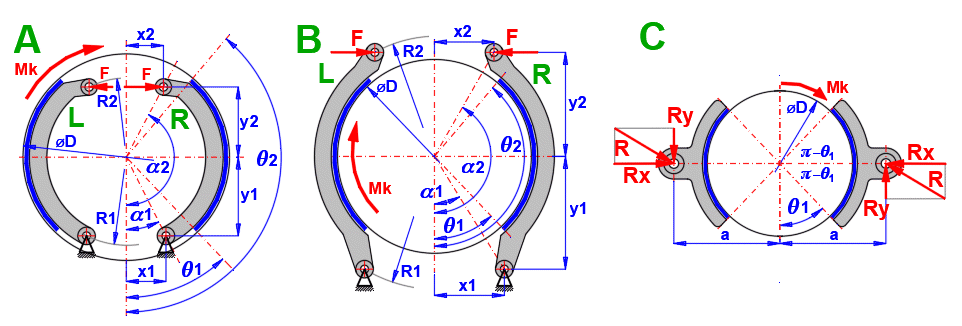### 4.1 Basic input values

Two basic parameters are required for the calculation and design of the brake/clutch. Torque Mk and friction coefficient f.
These parameters, together with the definition of the dimensions, lead to the calculation of the maximum pressure on the friction surface, the calculation of the actuating force and, knowing the energy and time parameters from , the calculation of the heating.

### 4.2 Braking/transfer torque

In the green field is the moment Mk from paragraph . If you only need to solve the geometry for the specified torque Mk, uncheck the button on the right and enter the Mk to be transmitted by the brake/clutch. This breaks the connection with paragraph  and thus the warming and speed are not calculated (the results are greyed out).

### 4.3 Friction coefficient

By unchecking the check button on the right you can enter a custom value.
The recommended value is based on the selected friction material [1.44].

### 4.4 Friction segment definition

In this section you define the dimensions of the friction element. You can check the dimensions in the figure on the right.

### 4.5 Brake / clutch type

Select the desired  type from the list.

### 4.6 Number of trailing (L) / leading (R) shoes

Enter the number of trailing shoes (L) and leading shoes (R).
If both the leading (R) and trailing (L) shoes are used, their numbers must be identical. NL/NR = 1/1, 2/2, .....
It is also assumed that they are the same size and the same actuating force F.
If only the leading or trailing shoe is used, it is possible to choose any number of shoes of the same dimensions.

### 4.9, 4.10 Pin position (radius and angle) / (x, y coordinates)

Enter the position of the pin relative to the centre of the drum. You can switch the input method on the right. You can check the position in the figure on the right.

### 4.11, 4.12 Position of force (radius and angle) / (x, y coordinates)

Specify the position of the force relative to the centre of the drum. You can switch the input method on the right. You can check the position in the figure on the right.

### 4.13 Braking segment angle from / to

Enter the angle for the start and end of the braking segment. You can check the position in the figure on the right.

### 4.14 Distance of the caliper pin from the drum axis

The distance of the shoe pin axis from the drum axis (Figure C), which ensures zero frictional moment to the shoe pin axis. This ensures symmetrical lining wear.

### 4.15 Fill factor

Brake (clutch) linings are often attached with rivets. This coefficient expresses the reduction of the friction surface of the lining by the respective mounting holes.

For glued linings cF = 1.0 and for riveted linings cF ~ 0.90 - 0.95.

### 4.16 Contact area of one friction segment

It is the area over which the friction segment abuts the friction surface. It depends on the definition of the friction segment dimensions.

### 4.17 Sliding area for the friction segment

It is the area of the brake/clutch, which the friction segment is in contact with (indicated by a dashed line if visible on the outline).

### 4.18 Values for friction surfaces

The results are split for the leading (R) and trailing (L) braking shoes. When using R+L simultaneously, it is advisable to size the design for a more loaded (R) shoe.

### 4.27 Friction speed

Most friction material manufacturers also state the maximum value of friction speed that the material can handle. The recommended value is based on the choice of material [1.43].

### 4.28, 4.29 Maximum pressure in the trailing shoe (L), leading shoe (R)

It is the maximum pressure at the most heavily loaded point of the lining. Lining manufacturers usually state its maximum value (see recommended value on the right). If the recommended maximum value is exceeded, try to modify the geometry or the type of lining.

### 4.30 Heat flux density through the surface S''

Some manufacturers also provide a maximum recommended heat flux value q for friction segments.

### 4.31 Approximate temperature rise

The friction lining has a poor thermal conductivity lambda compared to the thermal conductivity of the materials in contact with the lining. (lambda of friction lining ~ 0.1, lambda for steel ~ 54). Therefore, it is assumed that all heat generated by friction is absorbed by the metal part of the brake/clutch.

This indicative calculation uses only the weight of the disc (cone, drum) m. Ssee the calculation below. The calculation is correct if the disc is the energy recipient (typically a disc brake).

For other design arrangements (typically a clutch), the frictional energy is absorbed, for example, by the clutch housing, and the result is obviously invalid.

Note: The calculation includes one braking/running cycle. For a more detailed analysis use paragraph .

### 4.32 Friction surface warming

It is assumed that the braking/clutch engagement process is short. Therefore, no cooling during this process is considered. The calculation of the friction surface warming is based on this assumption, where only the heat transfer to the disc (drum, housing, etc.) is considered based on the thermal conductivity of the material.

### 4.33 Finding a solution (GoalSeek)

Problems like:
- How to change the dimensions to reach the allowed pmax
- How can the brake/clutch be loaded to achieve the allowed pmax
- What can be the maximum force Fn'
and others can be easily solved using the excel function "GoalSeek".

For the most common tasks the inputs to this function and its call are predefined.

After selecting the query and entering the desired value, the "Run" button will start the function execution.

You can return to the original value of the changed parameter by pressing the "Back" button. (The functionality is time-limited to prevent unwanted overwriting of values).

###### Note: The function does not control the inputs and results of the solution. Therefore, it is possible that no solution exists for the specified request. In this case, set the original value of themodified parameter and re-evaluate your requirements.

Example:
You want to calculate the friction segment angle for a disc brake that will provide the required maximum pressure "pmax". It is clear that the friction segment angle can only be within a certain range, but there is no limit to the pmax requirement. So a requirement for a large change in pmax can lead to a completely meaningless alpha segment angle.

### 4.34 Selection of the requirement

Select the problem you want to solve from the list.

### 4.35 Required parameter value

Enter the desired value to be reached by changing the input parameter and call the "Solution search" function using the button on the right.

### 4.36 Brake drum calculation

The dimensional parameters are estimated on the basis of the previous data. After unchecking the check button on the right, you can enter your own dimensions (see figure).

The mass value is used to calculate ΔT1.

The area value is used for the cooling calculation in paragraph .

### 4.39 Surface

The value of surface S' is used as a default for the calculation of warming in paragraph  and is not used in this paragraph.

The area S' is calculated according to the figure.## Band brakes /clutches 

The friction surface consists of a belt that is wrapped around the brake drum. It is used in industrial applications, simple design. It is suitable for manual operation when high braking performance can be achieved with relatively low operating force.### 5.1 Basic input values

Two basic parameters are required for the calculation and design of the brake/clutch. Torque Mk and friction coefficient f.
These parameters, together with the definition of the dimensions, lead to the calculation of the maximum pressure on the friction surface, the calculation of the actuating force and, knowing the energy and time parameters from , the calculation of the heating.

### 5.2 Braking/transfer torque

In the green field is the moment Mk from paragraph . If you only need to solve the geometry for the specified torque Mk, uncheck the button on the right and enter the Mk to be transmitted by the brake/clutch. This breaks the connection with paragraph  and thus the warming and speed are not calculated (the results are greyed out).

### 5.3 Friction coefficient

By unchecking the check button on the right you can enter a custom value.
The recommended value is based on the selected friction material [1.44].

### 5.4 Friction segment definition

In this section you define the dimensions of the friction element. You can check the dimensions in the figure on the right.

### 5.9 Contact area of one friction segment

It is the area over which the friction segment abuts the friction surface. It depends on the definition of the friction segment dimensions.

### 5.10 Sliding area for the friction segment

It is the area of the brake/clutch, which the friction segment is in contact with (indicated by a dashed line if visible on the outline).

### 5.11 Values for one band

The results determine the tensile force for one band F1 and F2 that is required to provide the required friction moment Mk.

### 5.15 Friction speed

Most friction material manufacturers also state the maximum value of friction speed that the material can handle. The recommended value is based on the choice of material [1.43].

### 5.16 Maximum pressure

It is the maximum pressure at the most heavily loaded point of the lining. Lining manufacturers usually state its maximum value (see recommended value on the right). If the recommended maximum value is exceeded, try to modify the geometry or the type of lining.

### 5.17 Heat flux density through the surface S''

Some manufacturers also provide a maximum recommended heat flux value q for friction segments.

### 5.18 Approximate temperature rise

The friction lining has a poor thermal conductivity lambda compared to the thermal conductivity of the materials in contact with the lining. (lambda of friction lining ~ 0.1, lambda for steel ~ 54). Therefore, it is assumed that all heat generated by friction is absorbed by the metal part of the brake/clutch.

This indicative calculation uses only the weight of the disc (cone, drum) m. Ssee the calculation below. The calculation is correct if the disc is the energy recipient (typically a disc brake).

For other design arrangements (typically a clutch), the frictional energy is absorbed, for example, by the clutch housing, and the result is obviously invalid.

Note: The calculation includes one braking/running cycle. For a more detailed analysis use paragraph .

### 5.19 Friction surface warming

It is assumed that the braking/clutch engagement process is short. Therefore, no cooling during this process is considered. The calculation of the friction surface warming is based on this assumption, where only the heat transfer to the disc (drum, housing, etc.) is considered based on the thermal conductivity of the material.

### 5.20 Finding a solution (GoalSeek)

Problems like:
- How to change the dimensions to reach the allowed pmax
- How can the brake/clutch be loaded to achieve the allowed pmax
- What can be the maximum force Fn'
and others can be easily solved using the excel function "GoalSeek".

For the most common tasks the inputs to this function and its call are predefined.

After selecting the query and entering the desired value, the "Run" button will start the function execution.

You can return to the original value of the changed parameter by pressing the "Back" button. (The functionality is time-limited to prevent unwanted overwriting of values).

###### Note: The function does not control the inputs and results of the solution. Therefore, it is possible that no solution exists for the specified request. In this case, set the original value of themodified parameter and re-evaluate your requirements.

Example:
You want to calculate the friction segment angle for a disc brake that will provide the required maximum pressure "pmax". It is clear that the friction segment angle can only be within a certain range, but there is no limit to the pmax requirement. So a requirement for a large change in pmax can lead to a completely meaningless alpha segment angle.

### 5.21 Selection of the requirement

Select the problem you want to solve from the list.

### 5.22 Required parameter value

Enter the desired value to be reached by changing the input parameter and call the "Solution search" function using the button on the right.

### 5.23 Calculation of the force F for different types of structure

The most commonly used structural arrangement is shown in the figure.Select the type of structure, the sense of rotation of the drum and enter the desired dimensions. The result is the control force F.

#### 1;2. Simple band brake

Use: For one direction of rotation, manual operation.

#### 3. Differential band brake

Use: For one direction of rotation, manual operation.
Advantage: A relatively small force F can be used to brake a large torque mornent. For a = b . e^(f*alpha), F = 0. But braking would be jerky, unsatisfactory.
Therefore, a > 2.5 * b is usually preferred.

#### 4. Additive differential band brake

Use: For both directions of rotation, for a = b, F is the same for both directions.

### 5.30 Brake drum calculation

The dimensional parameters are estimated on the basis of the previous data. After unchecking the check button on the right, you can enter your own dimensions (see figure).

The mass value is used to calculate ΔT1.

The area value is used for the cooling calculation in paragraph .

### 5.33 Surface

The value of surface S' is used as a default for the calculation of warming in paragraph  and is not used in this paragraph.

The area S' is calculated according to the figure.This chapter provides some additional calculations for determining brake/clutch loads and for warming calculation.

## Calculation of kinetic energy of rotating and moving mass 

The primary role of the brake/clutch is to stop/accelerate a defined mass (To remove/add kinetic energy Ek). In the vast majority of cases, these are the two basic tasks.
1. Brake / accelerate rotating parts
2. Brake / accelerate directly moving components
3. Combination of the above tasks

The calculations in this paragraph allow defining 5 calculations for each task. Check / uncheck the check button in the header to add / remove the sum of Ek to/from the final result on line [6.6].

### 6.1 Kinetic energy of rotating masses 1

A common problem in Ek calculation is the solution of a rotating cylinder. Give the dimensions and speed as shown in the figure.

### 6.2 Kinetic energy of rotating masses 2

The mass of the rotating component and the radius of inertia are sometimes given. You can use this table to calculate Ek.

### 6.3 Kinetic energy of rotating masses 3

The moment of inertia is often given for rotating components. In this case, use this table to calculate Ek.

### 6.4 Kinetic energy of linearly moving masses

To calculate Ek, enter the mass and velocity of the moving parts. If you need to convert linear motion to rotational speed, enter the diameter of the wheel in the column marked D.

### 6.7 Move value to [1.4] or [1.15]

Depending on the setting of the calculation type [1.2], the Ek value will be transferred to [1.4] (brakes) or [1.15] (clutches) when the button is pressed.

## Calculation of brake / clutch load torque 

In most cases, in addition to the kinetic energy (see paragraph 6), the brake/clutch system is also loaded with an additional torque, for example from:
- Frictional force of the bearings
- Frictional force of the mechanisms
- Movement on an inclined plane
- Aerodynamic drag
- etc.

The above calculations include the most commonly solved problems.

The value of the load moment ML can be transferred to paragraph  by pressing the ">> ML" button.

### 7.1 Vehicle movement

Calculation of the vehicle load/unload moment due to rolling resistance and movement on an inclined plane. Enter the required parameter values in the calculation.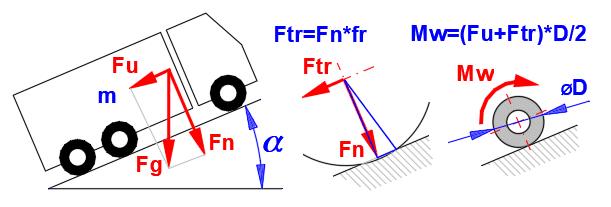### 7.6 Traction coefficient

Asphalt / Tire (truck)…(0.006-0.010)
Asphalt / Tire (passenger car)…(0.010-0.015)
Asphalt / Tire (motorcycle)…(0.010-0.015)
Asphalt / Tire (bike)…(0.002-0.005)
Pavement / Tire…(0.015-0.030)
Sand and gravel / Tire…(0.040-0.080)
Rail / Steel wheel…(0.001-0.002)

### 7.16, 7.41 Move value to [1.5] or [1.16]

Depending on the setting of the calculation type [1.2], the Ek value will be transferred to [1.5] (brakes) or [1.16] (clutches) when the button is pressed.

### 7.17 Elevator, crane

The additional loading/lightening moment depends on the weight of the lifted equipment . Enter the required parameter values in the calculation.### 7.26 Move value to [1.5] or [1.16]

Depending on the setting of the calculation type [1.2], the Ek value will be transferred to [1.5] (brakes) or [1.16] (clutches) when the button is pressed.

### 7.27 Movement on inclined plane

The additional loading/unloading moment depends on the mass, the coefficient of friction f and the angle of the inclined plane. Enter the required parameter values in the calculation.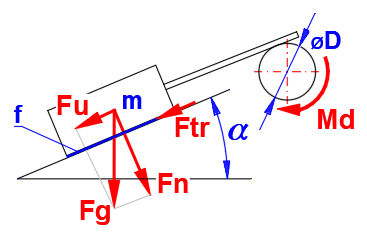### 7.30 Friction coefficient

=======Dynamic (v>0), Clean, Dry ========
Hardened steel - Hardened steel (0.1)
Hardened steel - Cast iron (0.13-0.27)
Hardened steel - Phosphore bronze (0.15)
Hardened steel - Wood (oak) (0.4-0.5)
Cast iron - Cast iron (0.15-0.2)
Cast iron - Phosphore bronze (0.15-0.2)
Cast iron - Wood (oak) (0.3-0.5)
Phosphore bronze - Phosphore bronze (0.2)
Leather - Cast iron (0.56)
Rubber - Cast iron (0.8)
Rubber - Concrete (0.7)
Asbestos (textile) - Steel (0.3-0.35)
Nylon - Steel (0.3)
Polystyrene - Steel (0.5)
=======Dynamic (v>0), Lubricated ========
Hardened steel - Hardened steel (0.05-0.1)
Hardened steel - Cast iron (0.05-0.15)
Hardened steel - Phosphore bronze (0.09-0.15)
Hardened steel - Wood (oak) (0.08)
Cast iron - Cast iron (0.07-0.1)
Cast iron - Phosphore bronze (0.07-0.1)
Cast iron - Wood (oak) (0.2)
Phosphore bronze - Phosphore bronze (0.07-0.1)
Leather - Cast iron (0.15-0.35)
Rubber - Cast iron (0.5)
Rubber - Concrete (0.3-0.5)
Asbestos (textile) - Steel (0.08-0.12)

## Calculation of brake / clutch warming 

During both braking and starting, kinetic energy is converted into heat when the friction surfaces come into contact. The preceding paragraphs give the relationships for calculating the maximum torque and maximum pressure on the friction surfaces for the specified brake/clutch dimensions. Even if the maximum torque is not transmitted and the maximum allowable pressure is not exceeded, the brake/clutch may be destroyed by excessive heat.

###### Example: Starting the car up a hill. Although the clutch is designed for this case (transferring more load), the driver can (incorrectly) compensate for the load by keeping the clutch engaged for a long time. In this case, frictional heat is continuously supplied to the clutch system and can damage the clutch when the limits are exceeded.

In this paragraph you can:
- Solve brake/clutch warming during single braking/clutch engagement.
- Simulate repeated brake/clutch application.

### 8.1 Dimensions and material parameters of the disc, drum, case...

After the selection of an option from the list [8.2], the surface size and weight values from the corresponding paragraph of the brake/clutch type [2,3,4,5] are filled in.
However, for a reasonable calculation (estimation) of the thermal characteristic, it is necessary to determine the surface area and mass as accurately as possible with respect to the brake/clutch design used.

As an example, consider the difference between a disc brake and a disc clutch.

Disc brake:
Consists of a metal disc that is braked by friction pads. All the heat is stored in the metal disc, which transfers the heat to the surrounding air. The values of area and mass from paragraph  can be used to calculate the heat.

Disc coupling:
It is created by a thin disc with a friction lining that engages the metal structure of the coupling. All the heat is thus stored in the coupling body. The area and mass parameters are completely different from those proposed in paragraph  and custom values must be entered.

To enter your own values, uncheck the check button on the right.

### 8.5 Specific heat capacity

The value is preset based on the material selection [1.54].

### 8.6 Single braking / clutch engagement

The energy values are transferred according to the choice of the type of calculation in paragraph . You can enter your own values by unchecking the check button on the right.

Values from [8.4, 8.5, 8.7] are used to calculate the temperature rise.

### 8.8 Temperature rise per cycle

One cycle of braking/clutch engagement will result in an approximate temperature rise of ΔT. The value is approximate, for a more detailed description please refer to the theoretical part of the help.

### 8.9 Repeated braking / clutch engagement (iterative calculation)

In this calculation, 100 steps of repeated braking/clutch engagement are simulated with a specified time interval [8.13]. For each step, the corresponding heat transfer coefficient alpha is calculated, which is then used to calculate the temperatures of the next step. In most cases, this procedure is satisfactory and converges quickly to a steady state temperature.

The temperature progression is shown in Figure B [8.25]. The x-coordinate is in minutes.

In the case of large brake/clutch thermal inertia (large mass), 100 iteration steps may be insufficient. This case can be recognized from graph B, where the curve does not converge to the steady state temperature Max/Min. In this case, use the cumulative calculation [8.17].

### 8.10 Air temperature

Enter the ambient air temperature.

### 8.11 Air velocity

The air velocity around the brake/clutch body significantly influences the heat transfer.

Therefore, try to estimate this value as accurately as possible.

### 8.12 Braking / clutch engagement time

The value is preset based on the calculation from . You can enter your own value by unchecking the check button on the right.

### 8.13 Time interval

Enter the time interval between repeated brake/clutch applications.

### 8.16 Maximum temperature

In Graph B you can usually see a gradual convergence to a steady state Min/ Max value.

The Min/ Max value is taken from the last step (#100) of the sequential iteration.

### 8.17 Repeated braking/clutch engagement (cumulative calculation)

This calculation estimates the total temperature increase. From this increase, the corresponding heat transfer coefficient alpha is calculated and used for the overall warming calculation.

### 8.18 Estimation of temperature rise

By unchecking the check button on the right, you can enter your estimate of the temperature rise. However, in most cases this is unnecessary and the recommended value in the green box is accurate enough.

### 8.22 Maximum temperature

Maximum brake/clutch temperature for an unlimited number of brake/clutch cycles.

### 8.24 Cycle sequence number:

You can enter the cycle number directly or set it with the slider on the right. The temperature progression of the selected cycle is shown in the graph.

## Example 1 - Calculation of a car brake

Design of a disc brake on the front axle of a passenger car + temperature analysis.### 1. Input parameters

Car weight: m = 1600 kg
Initial speed v1 = 180 km/h (50 m/s)
End speed v2 = 0
Average deceleration a = -7 m/s^2
Wheel diameter = 620 mm
Distribution of braking force 70% front 30% rear axle.
Repeated braking 180->0 km-h with a period of 180s

Braking time:
t = v1 / a; t = 50 / 7 => t = 7.14 s
v1-Initial velocity; a-Acceleration / Deceleration; t-time

Calculation assumptions:
- Aerodynamic drag is not included
- No wheel slip
- Linear progression

### Calculation procedure:

In paragraph  select the calculation units [1.1] and the brake calculation [1.2]

First, it is necessary to solve the brake load.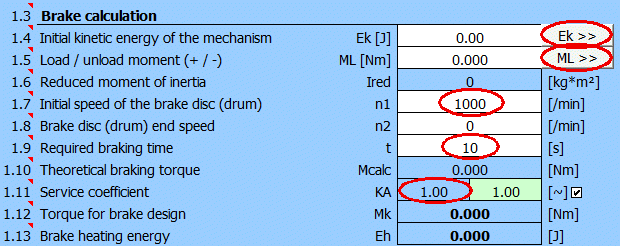To calculate the kinetic energy Ek, use paragraph , pressing the button "Ek >>".

Ek calculation for one braking disc. Front axle 70% load, one wheel => 0.7 / 2 = 0.35.
For passenger cars use a coefficient of 1.05 (which includes rotating braked masses such as wheels, shafts, gears, etc...).
First column 0.35 x 1.05 = 0.3675.
Resulting Ek=735000 [J] and for a wheel diameter of 620mm => speed 1540 [/min].To calculate the additional ML load moment, use paragraph , press the "ML >>" button.

We will distribute the total mass of the vehicle on one wheel of the front axle according to the previous consideration m=1600*0.35=560 [kg].
After entering the remaining data, we obtain the additional load moment 123 [Nm] and we will transfer it to paragraph .After filling in all the data and selecting KA=1.1, we have defined the parameters for calculating the dimensions of the disc brake (t, Mk, Eh).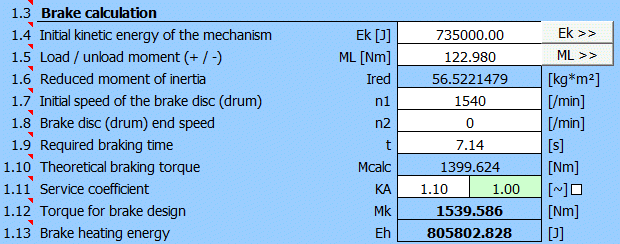#### Calculation of brake dimensions

It is advisable to define (select) the brake lining material and brake disc material in paragraph  beforehand.After switching to the paragraph for calculating the disc brake/clutch , set the initial parameters [2.1-2.7].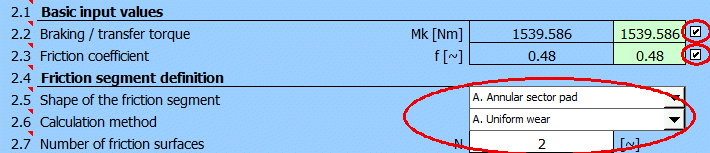Select friction segment angle alpha [2.11] = 80°.

It is likely that the value of the maximum pressure pmax [2.24] will either exceed the permissible value from [1.48] or be too low and the brake potential will not be used.

Therefore, select the "Goal Seek" (03.) for pmax on [2.29], enter the desired value of 2.5 [MPa] (see selected material) and press the "Run" button.
The minimum outer radius of the friction segment to achieve the specified pressure pmax=2.5 [MPa] will be searched.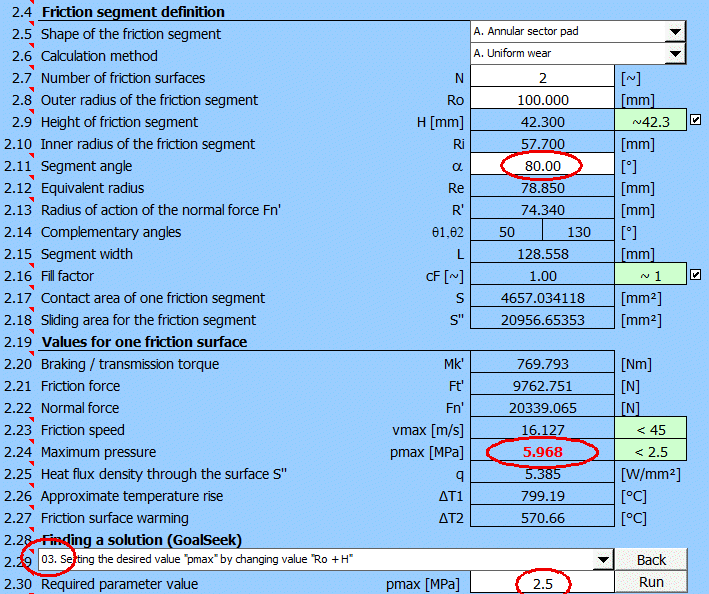The Goel Seek will provide these results:After a suitable choice of Ro and H values, the design of the disc brake will look as follows.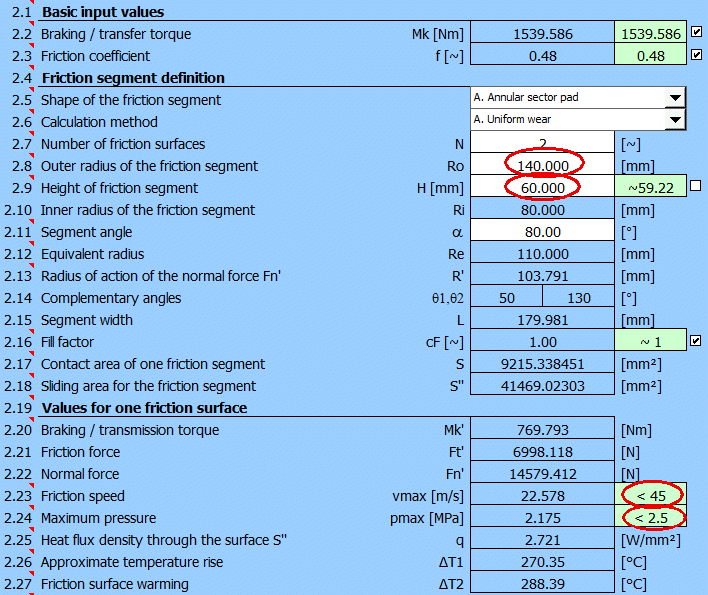The design meets the basic requirements for friction speed and lining pressure while meeting the braking torque requirement of Mk.

#### Temperature analysis

A thermal analysis should be an integral part of the brake design. The design of the brake disc dimensions is included in paragraph . These are necessary for the analysis.
For this example, use the preset (estimated) values.After moving to paragraph , set the parameter values as follows:

- [8.3] The disk parameters from the selected disk brake calculation  are used
- [8.7] The braking energy from paragraph  is used
Enter the air temperature, the air velocity (estimated to be half the vehicle speed at the start of braking) and the desired time interval between braking.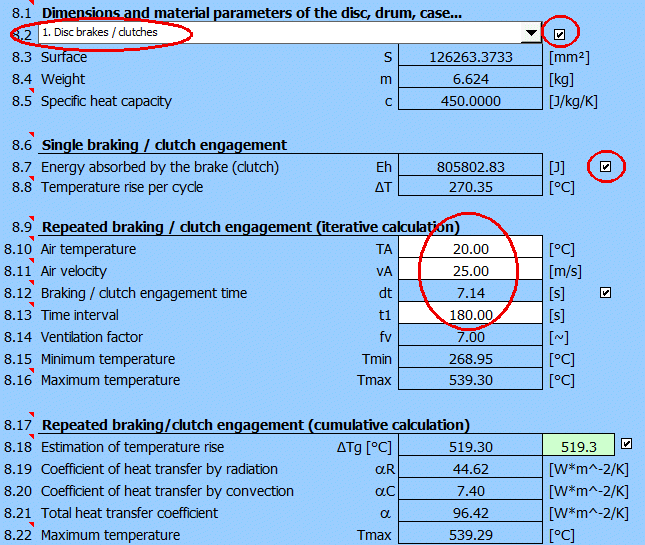The result is a graph of the temperature rise and fall between each braking cycle.## Example 2 - Calculation of a single-disc clutch of a passenger car

Design of a disc clutch of a passenger car.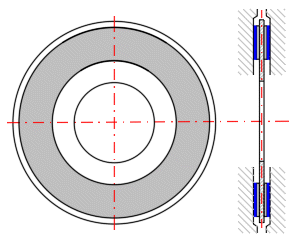### 1. Input requirements

Car weight: m = 1600 kg
Engine power diagramEstimated from the power chart:
- Selected speed for starting = 2500 [/min]
- Motor power for 2500 [/min] = 60kW

Initial speed v1 = 0
Final speed v2 = 25 km/h (7 m/s) for clutch speed 2500 rpm in 1st gear
Wheel diameter = 620 mm

Calculation assumptions and simplifications:
- Aerodynamic drag is not included
- There is no wheel slip
- Power and drive speed are assumed to be constant during clutch engagement

### Calculation procedure:

In paragraph  select the calculation units [1.1] and the coupling calculation [1.2].

First of all it is necessary to solve the load of the coupling.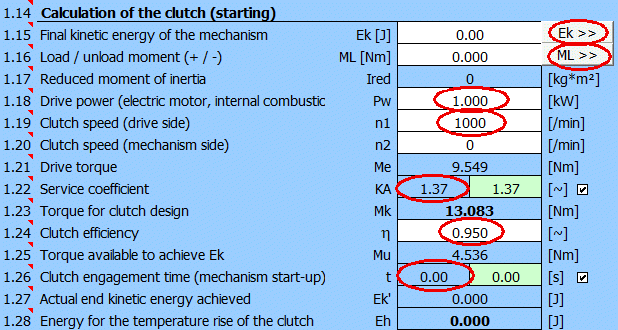To calculate the final kinetic energy Ek, use paragraph , press the button "Ek >>".

Calculation of the final Ek. Resulting Ek=39200 [J] and for wheel diameter 620mm => speed 215 [/min].To calculate the additional load moment ML, use paragraph , press the "ML >>" button.

Fill in weight, road grade, wheel diameter, wheel speed and clutch speed.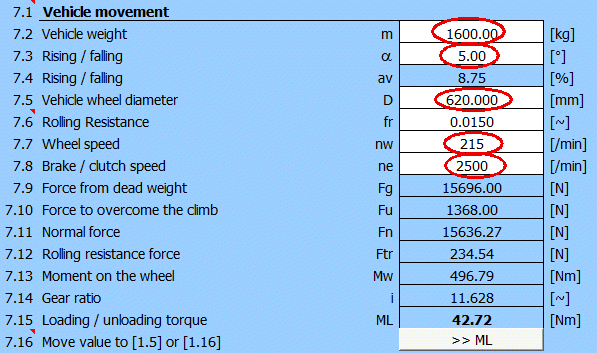After entering the data, you get an additional load torque of 42.7 [Nm]. Transfer the value to paragraph .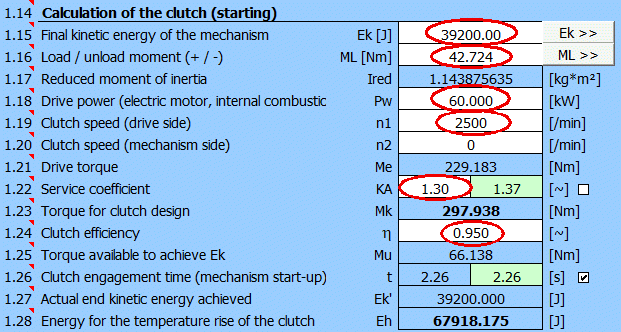After adding all the data and selecting KA=1.3, we have defined parameters for calculating the dimensions of the disc coupling (t, Mk, Eh).

#### Calculation of the dimensions of the disc coupling

Preliminarily, it is advisable to define (select) the material of the clutch lining and the material of the clutch housing in paragraph .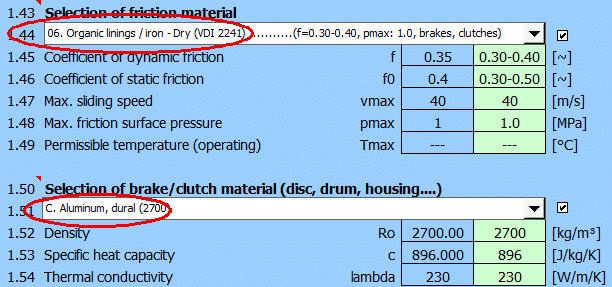After switching to the paragraph for calculating the disc brake/clutch , set the initial parameters [2.1-2.7].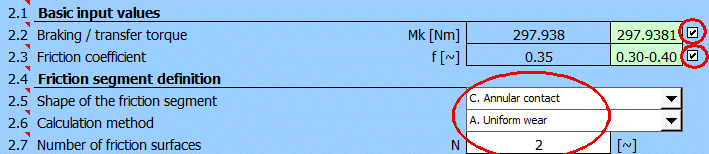It is likely that the value of the maximum pressure pmax [2.24] will either exceed the permissible value from [1.48] or be too low and thus the coupling potential will not be used.

Select the "Goal seek" (03.) for pmax on [2.29], enter a value of 1.0 [MPa] (see selected material) and press the "Run" button.
The minimum outer radius of the friction segment to achieve the specified pressure pmax=1.0 [MPa] will be searched.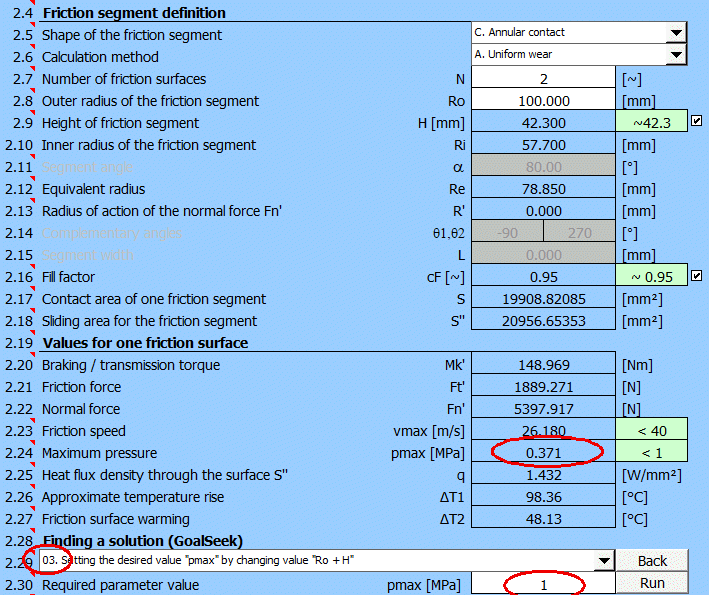The solution search will provide these results: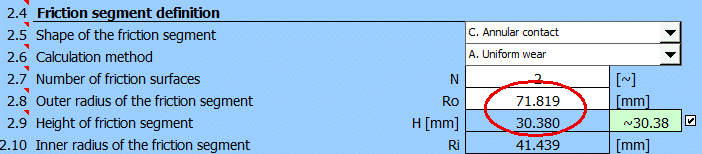After a suitable choice of Ro and H values, the design of the coupling will look as follows:The design meets the basic requirements for friction speed and lining pressure while meeting the braking torque requirement of Mk.

## Example 3 - Calculation of the actuating force for a drum brake, external lining

Calculation of the table of control force F and braking torque Mk for a defined brake.

### 1. Entry requirements

Brake dimensions according to the picture [mm].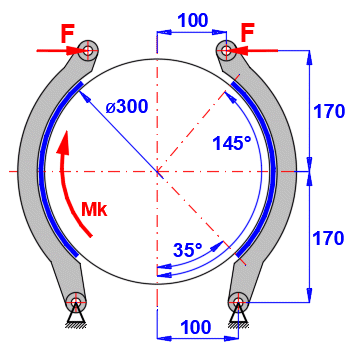Brake lining friction coefficient ................ f = 0.4 [~]
Brake lining width ................................ w = 100 [mm]
Glued lining => .................................. cF = 1.0 [~]
Maximum brake lining pressure ........ pmax = 0.5 [MPa]

Enter the brake dimensions as specified (uncheck the buttons on the right to enter).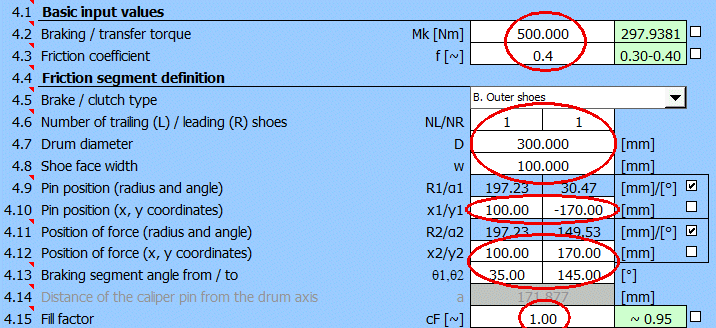Gradually change the torque Mk [Nm] in the range 300-1100.

Results table:

 ID Mk [Nm] F [N] pmaxR [MPa] 1 500 1932 0.24 2 600 2319 0.29 3 700 2705 0.34 4 800 3092 0.39 5 900 3478 0.43 6 1000 3865 0.48 7 1100 4251 0.53

Using the "Goal seek" function on [4.24-4.26] you can also find the maximum torque Mk and the maximum control force F for the maximum permissible pressure pmax=0.5 [MPa].

## Example 4 - Band brake

A band brake with the specified dimensions, find the maximum torque Mk and the actuating force F.

### 1. Input requirements

Brake dimensions as shown in the figure below: D=300mm, L=400mm, a=60mm, b=30mm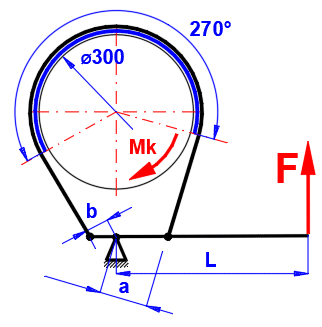Brake lining friction coefficient ................ f = 0.3 [~]
Brake lining width ................................ w = 100 [mm]
Maximum brake lining pressure ........ pmax = 0.3 [MPa]

Enter the brake dimensions as specified (uncheck the buttons on the right to enter).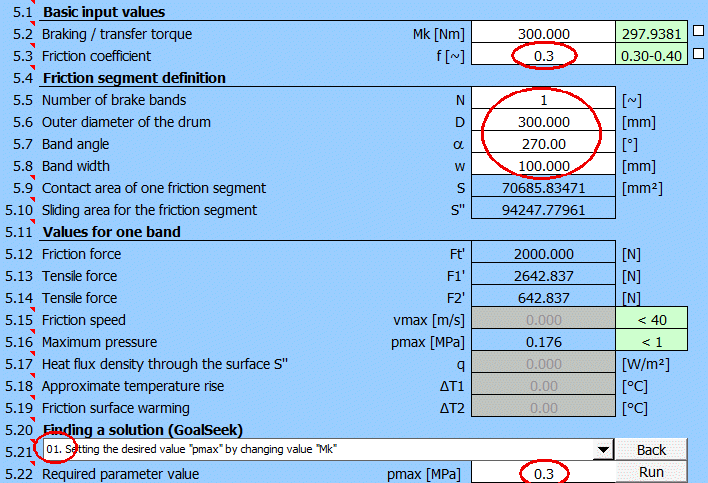Select [5.21] "Goal seek" (01.) for pmax, enter the desired value 0.3 [MPa] and press the "Run" button.
The moment Mk will be found to reach the specified pressure pmax = 0.3 [MPa].

After you select the type of control mechanism and enter dimensions, you will get the resulting solution: maximum torque Mk and control force F.## Setting calculations, change the language.

Information on setting of calculation parameters and setting of the language can be found in the document "Setting calculations, change the language".

## Workbook (calculation) modifications.

General information on how to modify and extend calculation workbooks is mentioned in the document "Workbook (calculation) modifications".

## List of Standards, literature list:

Litrature:
 Clutches and Brakes Design and Selection Second Edition (William C. Orthwein)
 Shigley’s Mechanical Engineering Design (Richard G. Budynas, J. Keith Nisbett)
 Textbook of Machine Design (R.S. KHURMI, J.K. GUPTA)
 Brake Design and Safety Third Edition (Rudolf Limpert)
 Roloff / Matek - Maschinenelemente, Normung, Berechnung, Gestaltung

Standards:
VDI 2241-Blatt 1
Schaitbare fremdbetätigte Reibkupplungen und -bremsen. Begriffe, Bauarten, Kennwerte, Berechnungen
Friction clutches and brakes Vocabulary, types, characteristic data, calculations

VDI 2241-Blatt 2
Schaitbare fremdbetätigte Reibkupplungen und -bremsen, Systembezogene Eigenschatten, Auswahlkriterien, Berechnungsbeispiele
Friction clutches and brakes Typ related properties, criteria for selection, examples of calculations

SAE J866
Friction Coefficient Identification and Environmental Marking System for Brake Linings

SAE J661
(R) BRAKE LINING QUALITY TEST PROCEDURE

Company cataloques: Ortlinghaus, Goizper, TAROX, FERODO...

^